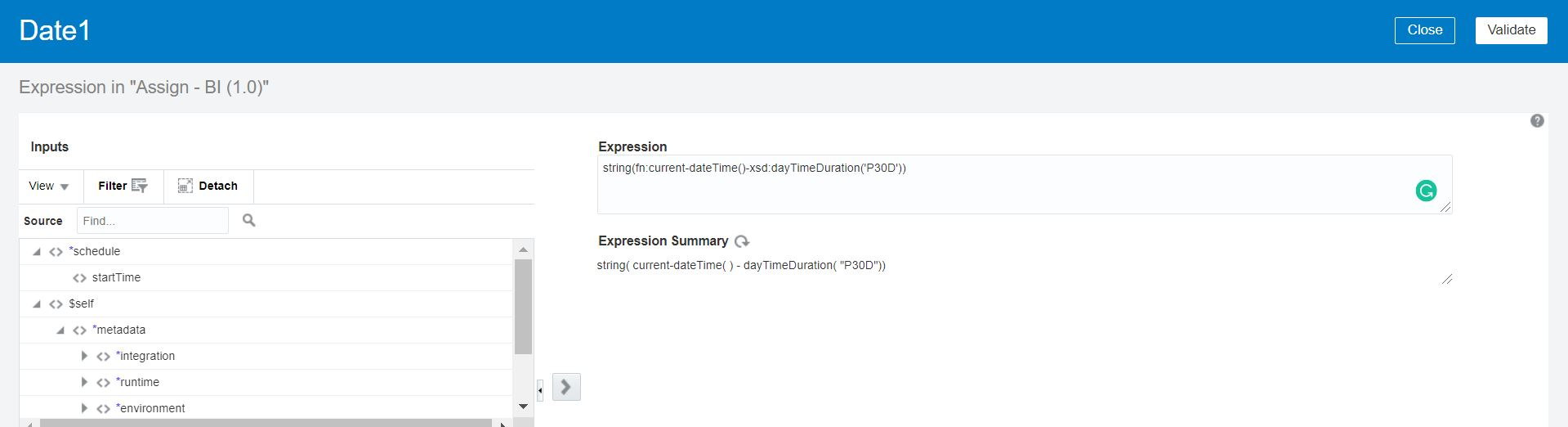There are scenarios where we wanted to add or subtract n number of days / hours / minutes in current date and time in Oracle Integration Cloud.For example:

• Subtract 30 days from Calendar date
• Add 5 hours 30 minutes in the current date
And so and so-on…
So to meet the requirement, we are writing this article that will help user to get the expected date and time.
In this article we’ll create a Scheduler integration and print the calculated date and time in logger.
Subtract 30 days from Calendar date
Drop an Assign activity below the Schedule activity and create a variable with name Date1. Open the Expression Builder.  Create the expression as below:
string(fn:current-dateTime()-xsd:dayTimeDuration(‘P30D’))
fn:current-DateTime function can be found under Function -> Date
xsd:dayTimeDuration function is under Functions -> ConversionNote: “30D” means 30 days. Notice the minus (-) operator between two functions which will minus the number of days.
Just Save the expression and come out from the Assign activity. Now add a Logger activity below the Assign activity and print variable Date1.
Run the scheduler and see the date should be printed which is 30 days prior of current date and time.
Add 05 hours 30 minutes in the current date
To add 05 hours and 30 minutes in current date and time, modify the previous variable Date1 and assign below expression
string(fn:current-dateTime()+xsd:dayTimeDuration(‘PT5H30M’))
Note: “5H30M” means 5 hours and 30 minutes. Notice the plus(+) operator between two functions which will plus the number of hours in current date and time.
Run the scheduler and see the date and time should be printed which is 5 hours 30 minutes ahead.
Similarly, we can add / subtract number of days or hours in current date and time.